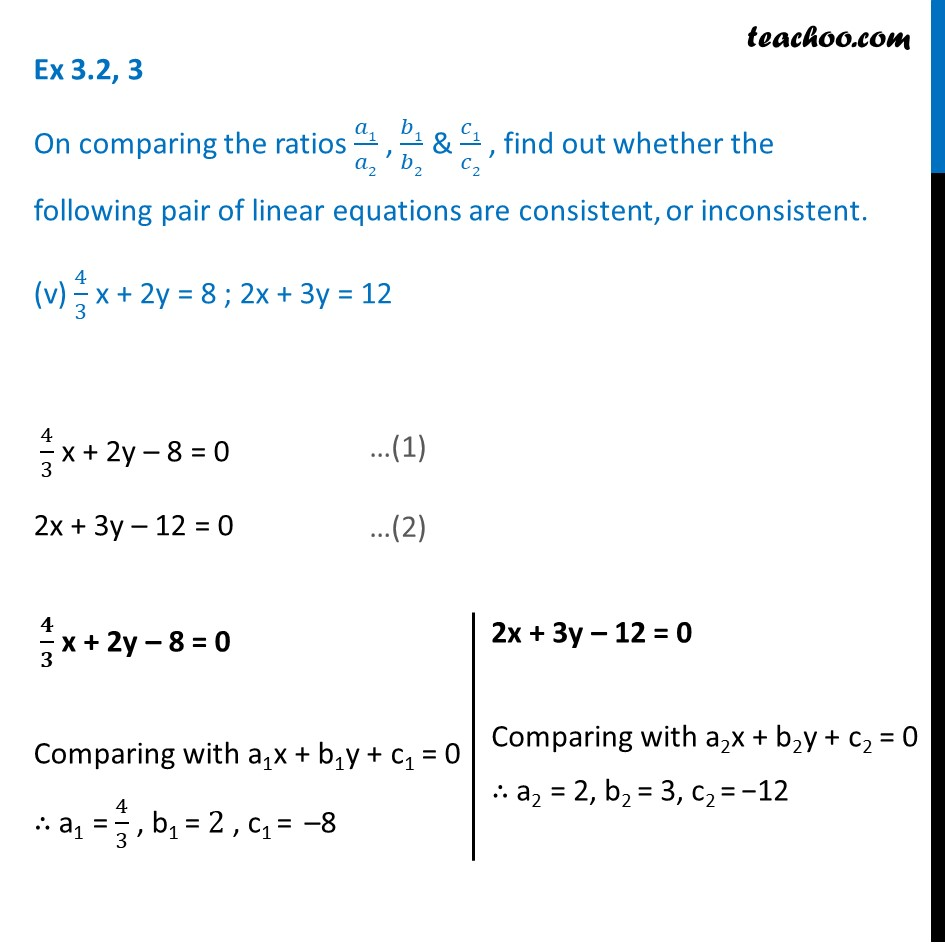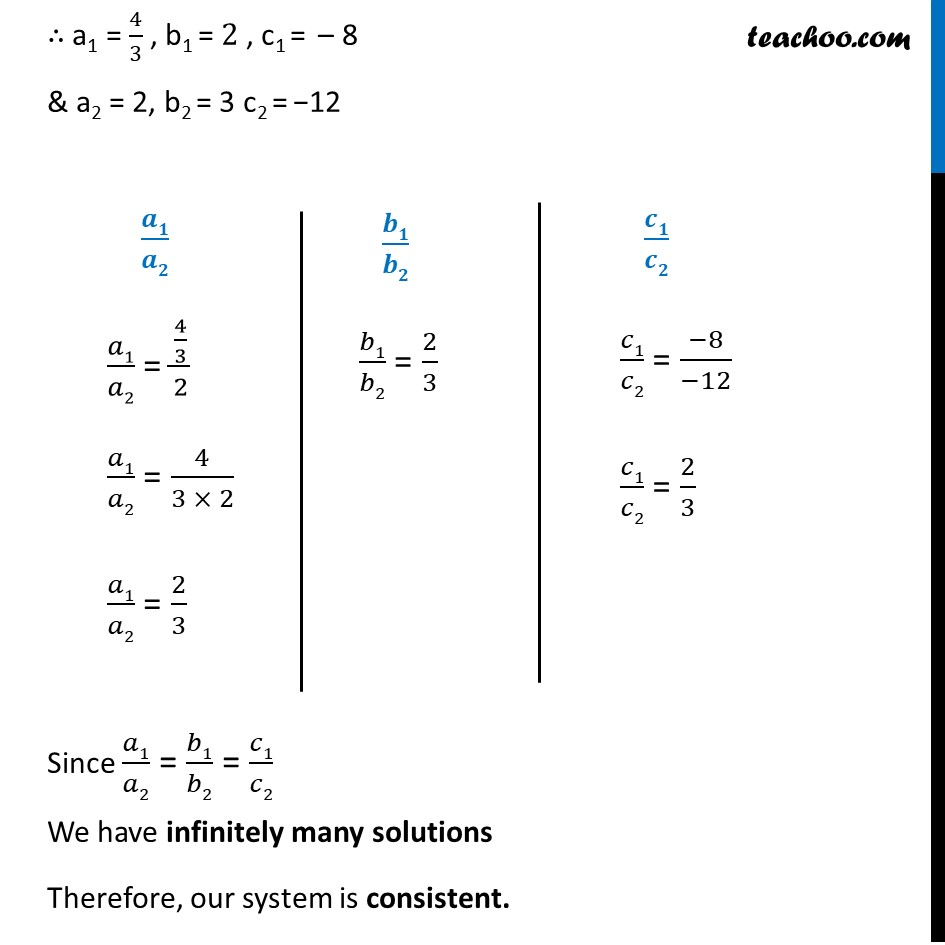1. Chapter 3 Class 10 Pair of Linear Equations in Two Variables (Term 1)
2. Serial order wise
3. Ex 3.2

Transcript

Ex 3.2, 3 On comparing the ratios 𝑎1/𝑎2 , 𝑏1/𝑏2 & 𝑐1/𝑐2 , find out whether the following pair of linear equations are consistent, or inconsistent. (v) 4/3 x + 2y = 8 ; 2x + 3y = 12 4/3 x + 2y – 8 = 0 2x + 3y – 12 = 0 𝟒/𝟑 x + 2y – 8 = 0 Comparing with a1x + b1y + c1 = 0 ∴ a1 = 4/3 , b1 = 2 , c1 = –8 2x + 3y – 12 = 0 Comparing with a2x + b2y + c2 = 0 ∴ a2 = 2, b2 = 3, c2 = −12 ∴ a1 = 4/3 , b1 = 2 , c1 = – 8 & a2 = 2, b2 = 3 c2 = −12 𝒂𝟏/𝒂𝟐 𝑎1/𝑎2 = (4/3)/2 𝑎1/𝑎2 = 4/(3 × 2) 𝑎1/𝑎2 = 2/3 𝒃𝟏/𝒃𝟐 𝑏1/𝑏2 = 2/3 𝒄𝟏/𝒄𝟐 𝑐1/𝑐2 = (−8)/(−12) 𝑐1/𝑐2 = 2/3 Since 𝑎1/𝑎2 = 𝑏1/𝑏2 = 𝑐1/𝑐2 We have infinitely many solutions Therefore, our system is consistent.